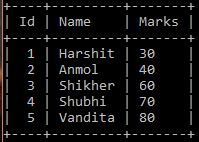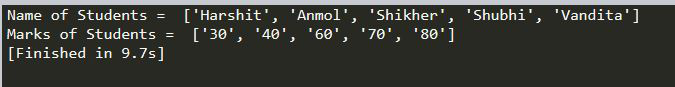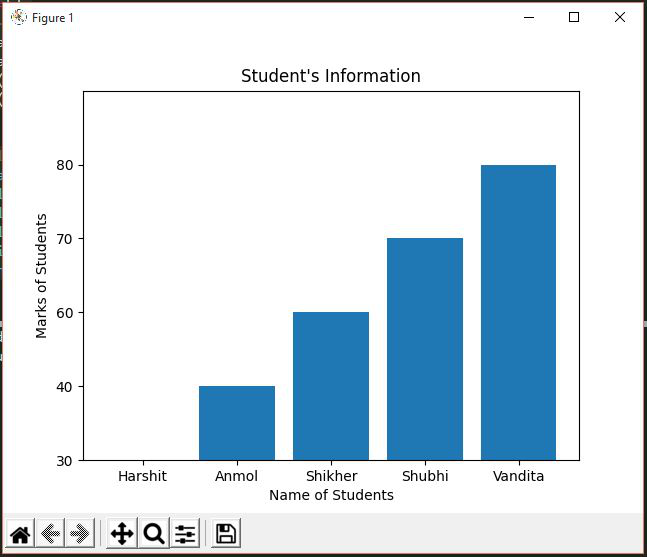Open in App
Not now

# How to visualize data from MySQL database by using Matplotlib in Python ?

• Difficulty Level : Basic
• Last Updated : 25 Jan, 2023

Prerequisites: Matplotlib in Python, MySQL

While working with Python we need to work with databases, they may be of different types like MySQL, SQLite, NoSQL, etc. In this article, we will be looking forward to how to connect MySQL databases using MySQL Connector/Python. MySQL Connector module of Python is used to connect MySQL databases with the Python programs, it does that using the Python Database API Specification v2.0 (PEP 249). It uses the Python standard library and has no dependencies.

In this article, we are going to discuss How to visualize data from the MySQL database by using matplotlib in Python. In order to perform this task, we just need to install a module name mysqlconnector which can be installed by using

`pip install mysqlconnector`

Now to use the matplotlib library in python we also need to install it. We can install it by using:

`pip install matplotlib`

In addition to these modules, we will also install another module which is numpy that will act as a utility module for efficient working of matplotlib. On the other hand, NumPy has enormous use in performing mathematical and logical operations on Arrays and dealing with mathematical computations.

Type the above commands in your command prompt of the Windows operating system to install the required modules.

Now to use the installed modules we have to import them in Python. This can be done as follows:

`import numpy as np `

Here np is simply an alias for numpy. In place of np we can take any name as we want. It is used so that we can write np in place of numpy.

`import matplotlib.pyplot as plt  `

matplotlib.pyplot is a collection of functions that make matplotlib work, importing it as plt means we can write plt in place of matplotlib.pyplot.

Steps to Connect MySQL database with Python:

• The first thing we need to do is to import mysqlconnector that we have installed earlier this can be done by writing:
`import mysql.connector`
• Now we can create a variable say mydb which is used to store the result of the connection. So we can connect MySQL with Python by using the connect() method which belongs to mysql.connector class this can be done as follows:

• As mentioned in the above piece of code that this connect() method requires some arguments that are as follows:
• host which can be your localhost or some other host.
• user which is no other than the username of mysql database.
• database which is the name of database from which the data is to be fetched.

At this point, we are done with the connection of MySQL database with Python. Now our intention is to fetch information from the database, so we create a variable say mycursor which will store the cursor of the current database. A cursor allows you to iterate a set of rows returned by a query and process each row to get the desired information.

`mycursor=mydb.cursor()`

Given a Student Record in MySQL database plot a graph between Student Name and Marks obtained by Students. To solve the above problem first we have to connect MySQL to Python.

Sample Table to be used:Now in order to obtain the desired query, we use execute() method of mycursor which will take SQL query as an argument and also we store the result of the query by using fetchall of mycursor this can be done as follows:

## Python3

 `mycursor.execute(``"select Name, Marks from student_marks"``)``result ``=` `mycursor.fetchall`

As you can see in the above query we are trying to fetch Student Name and Student Marks from the student_marks table. Now we store student Name and their respective Marks into two separate lists so that we can plot them in a bar graph.

## Python3

 `Names ``=` `[]``Marks ``=` `[]` `for` `i ``in` `mycursor:``    ``Names.append(i[``0``])``    ``Marks.append(i[``1``])` `print``(``"Name of Students = "``, Names)``print``(``"Marks of Students = "``, Marks)`

Visualizing Data using Matplotlib:

## Python3

 `# plt.bar to plot a bar graph``# with given values``plt.bar(Names, Marks)` `# Setting count of values in``# y-axis``plt.ylim(``0``, ``5``)` `# setting xlabel of graph``plt.xlabel(``"Name of Students"``)` `# setting ylabel of graph``plt.ylabel(``"Marks of Students"``)` `# setting tile of graph``plt.title(``"Student's Information"``)` `# show() method to display the graph``plt.show()`

Below is the full implementation of the above Approach:

## Python3

 `# Connecting to mysql database``import` `mysql.connector``import` `numpy as np``import` `matplotlib.pyplot as plt`  `mydb ``=` `mysql.connector.connect(host``=``"localhost"``,``                               ``user``=``"root"``,``                               ``password``=``"password"``,``                               ``database``=``"student_info"``)``mycursor ``=` `mydb.cursor()` `# Fecthing Data From mysql to my python progame``mycursor.execute(``"select Name, Marks from student_marks"``)``result ``=` `mycursor.fetchall` `Names ``=` `[]``Marks ``=` `[]` `for` `i ``in` `mycursor:``    ``Names.append(i[``0``])``    ``Marks.append(i[``1``])``    ` `print``(``"Name of Students = "``, Names)``print``(``"Marks of Students = "``, Marks)`  `# Visualizing Data using Matplotlib``plt.bar(Names, Marks)``plt.ylim(``0``, ``5``)``plt.xlabel(``"Name of Students"``)``plt.ylabel(``"Marks of Students"``)``plt.title(``"Student's Information"``)``plt.show()`

Output:My Personal Notes arrow_drop_up